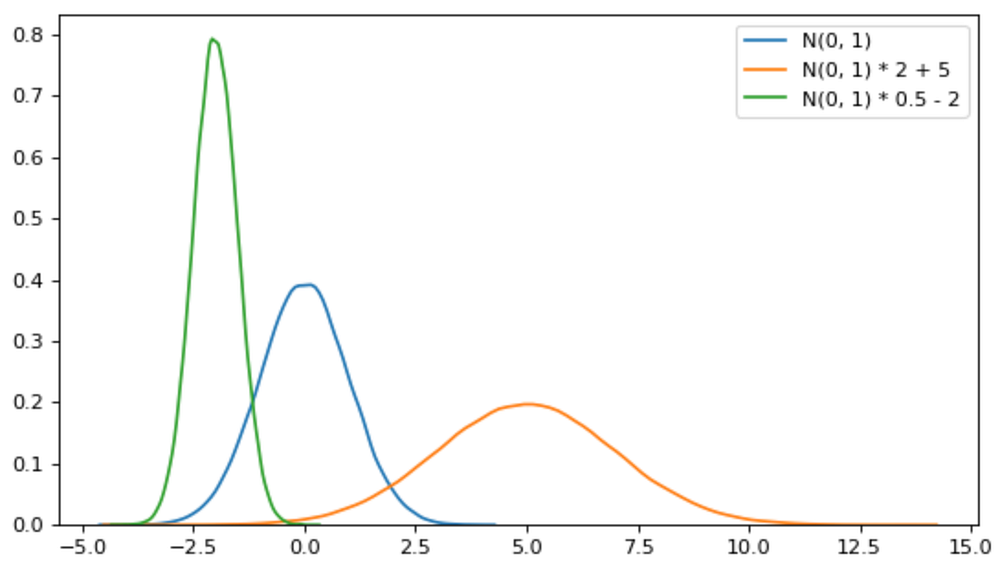# The reparameterization trick with code example

Original article was published on Deep Learning on Medium# The reparameterization trick with code example

First time I hear about this (well, actually first time it was readen…) I didn’t have any idea about what was it, but hey! it sounds pretty cool!

That’s the thing, you can’t backpropagate when you pick up random numbers. Randomness on a computer usually means reading the voltage of some component, using the internal clock… some interaction with the world. There is no way to calculate the derivative of that.

This happens if we use variational auto-encoders for example, and maybe with bayesian networks? Things like that. This happens when:

• We obtain a probability distribution. Another non typical example: noise layers for DQN.
• We sample from the distribution.
• We use the sampled value as the input for another layer.

Then at some point we want to backpropagate, how do we propagate through a random number? ¯\_(ツ)_/¯

## De-Standarization of the distribution

Say we have a normal distribution as N(10, 5), that means mean 10, standard deviation 5 (I prefer to read as location 10 and scale 5). It’s the same as doing:

N(0, 1) *5 + 10

Don’t you believe me? Here you have an example with numpy: